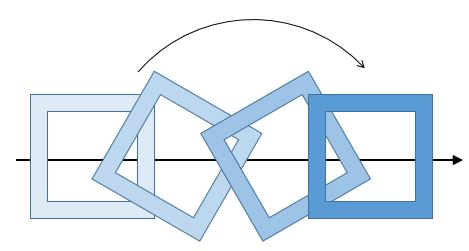# Rotational Kinetic Energy Of A SquareA frustrated painter grabs a wooden frame and throws it through his studio. As the frame flies through the air, it rotates about its central perpendicular axis. (See drawing for an idea of the shape and motion.)

The painter's wife, being a student of physics, observes that whenever a corner of the frame is at the bottom, it is instantaneously at rest.

What percentage of the frame's kinetic energy is rotational kinetic energy?

Assumptions and information

The frame and the hole in it are square and concentric. The side of the square hole is 90% of the side of the frame. The mass is uniformly distributed in the frame.

For a uniform square of side $a$ with a hole of side $b$, the moment of inertia about the perpendicular axis through the center is $I = \frac 1 6 m (a^2 + b^2.)$

Ignore the effect of gravity on the path of the frame; assume it is moving in a straight line.

×